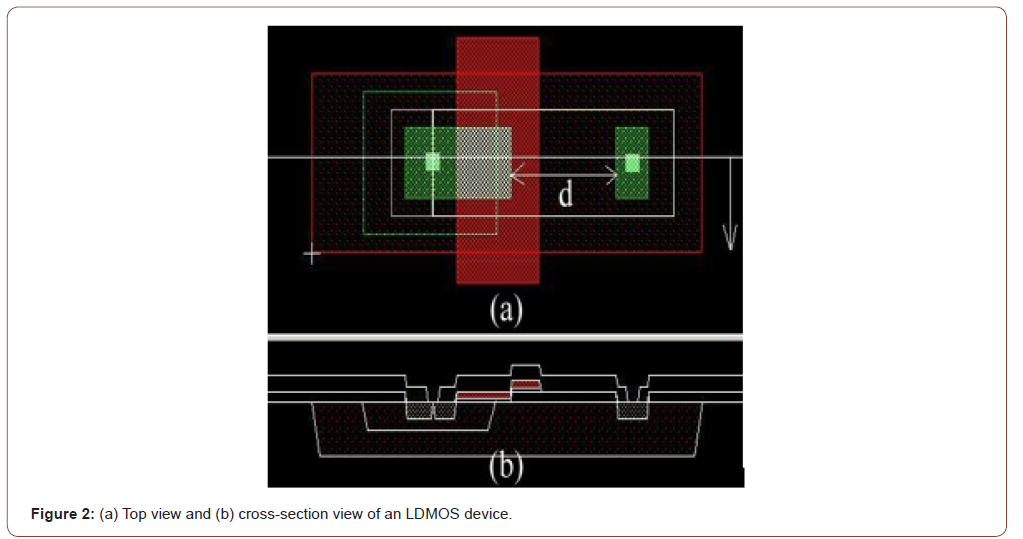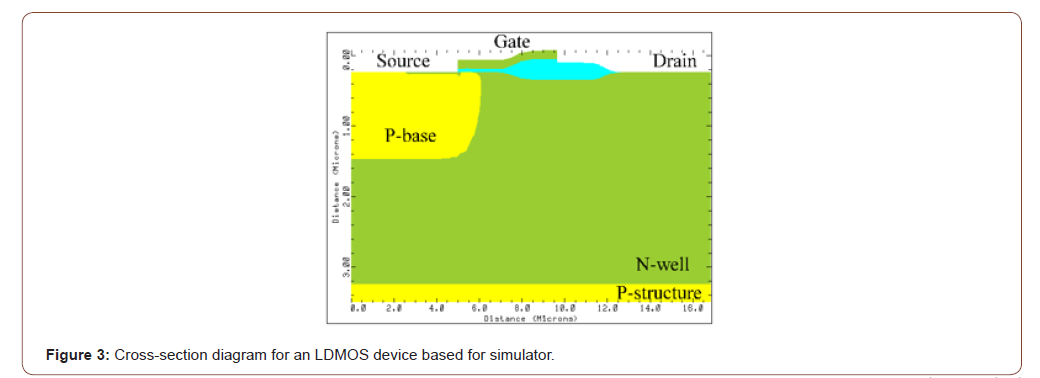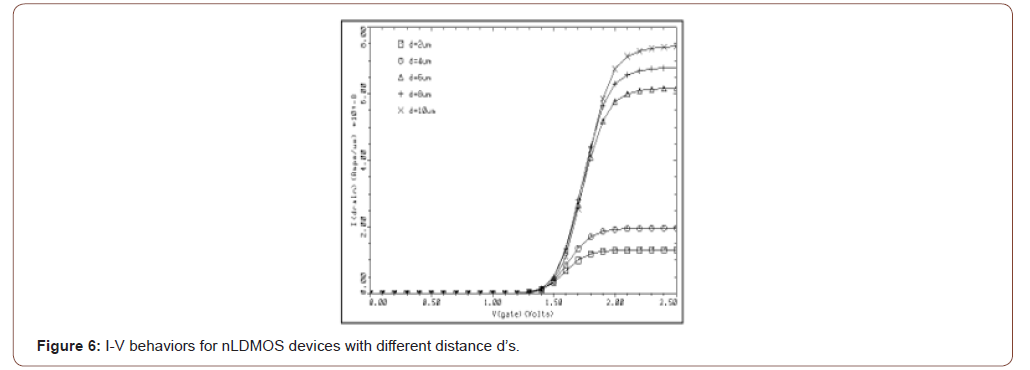# Optimized Design of the 100-V Silicon Based Power N-Channel LDMOS Transistor

Shen Li Chen* and SP Lee

Department of Electronic Engineering, National United University, Taiwan

Corresponding Author

Received Date: July 02, 2020;  Published Date: July 21, 2020

#### Abstract

In power integrated circuits (PICs), it is desirable to minimize the area of a power device region while maximizing its performances (i.e., higher breakdown voltage and lower on-resistance). Therefore, the area of a power device region mainly determines the total chip size and hence the cost. An optimized design of breakdown voltage and on-resistance in a power n-channel lateral-diffused MOSFET (nLDMOS) was investigated in this paper. Two-dimensional process and device simulators, such as the TSUPREM4 and Sentaurus EDA tools, will be used to predict the device characteristic behaviors. Eventually, it can be shown that a 100 V device will have an optimized breakdown voltage about 156.7 volts and onresistance Ron about 40.61 mΩ-cm2 under the Vgs-Vth= 5 V and LOCOS spacing d= 6 μm situations.

Keywords: Breakdown voltage; High Voltage (HV); Local Oxidation of Silicon (LOCOS); N-channel lateral-diffused MOSFET (nLDMOS); Onresistance

#### Introduction

A lateral double-diffused MOSFET (LDMOS) component has been widely used in smart power ICs, lighting, automotive system, and 5G communication applications [1-8]. It is with the advantage of its process compatible to VLSI process and easy to integrate with other CMOS devices. Consequently, it is very important to improve its electrical performance by optimizing their breakdown voltage and on-resistance. A cost effective and elegant method to utilize such a trade-off between on-resistance and breakdown voltage is to optimize the device physical dimension design.

For an n-channel LDMOS structure, the device is operated with a high positive voltage applied to the drain end. When the gate electrode is short-circuited to the source side, the device can support a large drain voltage across the P-base/N-drift layer junction. The breakdown voltage is dependent not only on the device structure, but also is affected by the physical dimension design of this device [9-15].

Meanwhile, when a positive bias is applied to the gate electrode, the surface channel of nLDMOS becomes conductive. At a low drain voltage, the current flow is essentially resistive, with the on-resistance determined by the sum of the source, channel, drift region, and drain-end resistances. The channel resistance decreases with increasing a gate bias, whereas the source/drain and drift region resistances remain a constant. Then, the total onresistance decreases with increasing a gate bias until it approaches a constant value. Under a large gate bias voltage, the channel resistance becomes smaller than that of the drift region resistance, and the device on-resistance becomes independent of gate bias. The total on-resistance is a measure of the current handling capability of the device because it determines the power dissipation during the current conduction. The on-resistance is defined as the slope of output characteristics in the linear region at low drain voltages. Furthermore, the on-resistance parameter is an important power MOSFET parameter in circuit designs.

#### Device Structure

Figure 1 shows the cross-section structure scheme of an LDMOS device, meanwhile, the top view of layout design and the corresponding cross-section of an LDMOS are presented in Figure 2. For a high-voltage LDMOS component, a field-oxide layer is fabricated near the drain side and used to improve the breakdown voltage. However, the Ron increased significantly due to an extra current path underneath this region. Then, in order to improve the Ron resistance [16-24], this LOCOS extra current path should be reduced. Unfortunately, by reducing the extra current path, the drain peak field is too close to the gate edge and the breakdown voltage will be significantly reduced.

In this paper, an optimized spacing distance underneath the field oxide dependence of high performance in the 100 V nLDMOS based on numerical simulators such as TSUPREM4 and Sentaurus will be investigated.

#### Process Simulation in an NLDMOS

The explored devices were processed by using a 100 V BCD process, and process simulations were performed by the TSUPREM4 simulator . One part of the cross-section diagram for an LDMOS device based on simulator is shown in Figure 3.A p-type substrate with σ= 15~25 Ω-cm is selected, and an HV N-well serves as the drift region. In the following process, phosphorus and boron ions were implanted into the HV N-well and P-base regions, respectively. And, then a high temperature process was executed and used to drive-in. Eventually, the field oxide thickness was set to be 6000 Å. The gate oxide thickness was 400 Å formed by a thermal SiO2 layer; an n+ polysilicon with 1250 Å thickness was used to as the gate electrode; and source/drain regions were fabricated by implanting arsenic atoms. Finally, the detailed process information is listed in Table 1.

Table 1:Process parameters of an nLDMOS device.#### Device Simulation in an NLDMOS

The two-dimensional numerical device simulator Sentaurus  is used to predict the device performance of nLDMOS devices. Here, the 2-D device simulator Sentaurus with TSUPREM4 resultant impurity data as the import file was used; the Sentaurus program solves the Poisson and continuity equations by numerical methods.

The breakdown voltage capability that is functioned of the distance d underneath the field oxide is shown in Figure 4 and 5. As expected, the breakdown voltage is increased with a longer distance d. Figure 6 and 7 are the plot of devices threshold voltages with different distance d’s. It is shown that the threshold voltage slightly decreased with the decreasing distance d value. Finally, the simulated Ron for nLDMOS devices with various distance d’s under a Vgs-Vth= 5V biased condition is illustrated in Figure 8 and 9. Increasing LOCOS spacing d, therefore, the current flow path increasing, an extra current path resulted in higher breakdown voltage and on-resistance values. Therefore, a “figure of merit (FOM)” index is defined asThen, the FOM values versus different distance d’s can be shown in Figure 10, as an nLDMOS is fabricated with a 6-μm spacing width underneath the field oxide, which provides a maximized FOM value in which the corresponding breakdown voltage is 156.7 V and on-resistance under the Vgs-Vth= 5V condition is 40.61mΩ-cm2. Therefore, the d= 6-μm spacing width is a best receipt in this 100 V nLDMOS fabrication by the LOCOS spacing adjustment.#### Conclusion

A high performance power device is essential for power integrated circuits. However, the simulation is an essential tool in the optimization of a complicated high-voltage MOSFET design. A combination of 2-D process and device simulations has been used to design and optimize the fabrication of a 100V n-channel lateraldiffused MOSFET (nLDMOS) device for high voltage applications. A high performance nLDMOS device has been proposed and achieved in this paper. A spacing “d” underneath the field oxide will affect the breakdown voltage and on-resistance eventually. Finally, when an nLDMOS is fabricated with a 6-μm LOCOS spacing underneath the field oxide, it provides a best condition in electrical behaviors, in which the breakdown voltage is 156.7V and on-resistance under the Vgs-Vth= 5V biased condition is 40.61 mΩ-cm2.

#### Acknowledgement

In this paper, authors would like to thank the Taiwan Semiconductor Research Institute in Taiwan for providing the process information and fabrication platform. And, authors would like to acknowledge the financial support of the Ministry of Science & Technology of Taiwan.

#### Conflict of Interest

No conflict of interest.

Article Details
Citation
Keywords
Scroll to
Scroll to Top